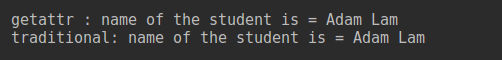# Python getattr()

Published on August 3, 2022By PankajWhile we believe that this content benefits our community, we have not yet thoroughly reviewed it. If you have any suggestions for improvements, please let us know by clicking the “report an issue“ button at the bottom of the tutorial.

In our previous tutorial we learned about python system command. In this tutorial we will be discussing about Python getattr() function.

## Python getattr() function

Python getattr() function is used to get the value of an object’s attribute and if no attribute of that object is found, default value is returned. Basically, returning the default value is the main reason why you may need to use Python getattr() function. So, before starting the tutorial, lets see the basic syntax of Python’s getattr() function.

``````getattr(object_name, attribute_name[, default_value])
``````

### Python getattr() example

In this section, we will learn how to access attribute values of an object using `getattr()` function. Suppose, we are writing a class named `Student`. The basic attributes of Student class are `student_id` and `student_name`. Now we will create an object of the class Student and access it’s attribute.

``````class Student:
student_id=""
student_name=""

# initial constructor to set the values
def __init__(self):
self.student_id = "101"

student = Student()
# get attribute values by using getattr() function
print('\ngetattr : name of the student is =', getattr(student, "student_name"))

# but you could access this like this
print('traditional: name of the student is =', student.student_name)
``````

So, the output will be like this### Python getattr() default value

In this section, we will use the python getattr() default value option. If you want to access any attribute that doesn’t belong to the object, then you can use the getattr() default value option. For example, if the `student_cgpa` attribute is not present for the student, then will show a default value. In the following example we will see example of default value. We will also learn what happens if the attribute is not present and we are not using the default value option.

``````class Student:
student_id=""
student_name=""

# initial constructor to set the values
def __init__(self):
self.student_id = "101"

student = Student()
# using default value option
print('Using default value : Cgpa of the student is =', getattr(student, "student_cgpa", 3.00))
# without using default value
try:
print('Without default value : Cgpa of the student is =', getattr(student, "student_cgpa"))
except AttributeError:

``````

So, after running the code you will get output like this

``````Using default value : Cgpa of the student is = 3.0
``````

Notice that `AttributeError` is raised when the default value is not provided while calling getattr() function.

### Reason for using Python getattr() function

The main reason to use python getattr() is that we can get the value by using the name of the attribute as String. So you can manually input the attribute name in your program from console. Again, if an attribute is not found you can set some default value, which enables us to complete some of our incomplete data. Also if your Student class is work in progress, then we can use getattr() function to complete other code. Once Student class has this attribute, it will automatically pick it up and not use the default value. So, that’s all about python getattr() function. If you have any query about this, please use the comment box below. Reference: Official Documentation

Thanks for learning with the DigitalOcean Community. Check out our offerings for compute, storage, networking, and managed databases.Pankaj

author

#### Still looking for an answer?

Ask a questionSearch for more help

JournalDev
DigitalOcean EmployeeApril 21, 2020

Hey man, I wonder why Python developers used getattr without passing a default argument instead of simply using the dot syntax… (found it in functools module source)

- Yeseen

JournalDev
DigitalOcean EmployeeJanuary 21, 2020

Nice It was clear in one go.

- shivam

JournalDev
DigitalOcean EmployeeAugust 26, 2019

The above brief explanation is very cool. Keep it up.

- Mr. Black Hawk